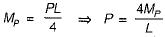Courses

# Plastic Analysis

## 10 Questions MCQ Test Mock Test Series for Civil Engineering (CE) GATE 2020 | Plastic Analysis

Description
This mock test of Plastic Analysis for Civil Engineering (CE) helps you for every Civil Engineering (CE) entrance exam. This contains 10 Multiple Choice Questions for Civil Engineering (CE) Plastic Analysis (mcq) to study with solutions a complete question bank. The solved questions answers in this Plastic Analysis quiz give you a good mix of easy questions and tough questions. Civil Engineering (CE) students definitely take this Plastic Analysis exercise for a better result in the exam. You can find other Plastic Analysis extra questions, long questions & short questions for Civil Engineering (CE) on EduRev as well by searching above.
QUESTION: 1

Solution:
QUESTION: 2

Solution:
QUESTION: 3

### In a plastic analysis of structures, the segment between any two successive plastic hinges is assumed to deform as

Solution:

The initial slopes and deflections of the beam do not affect the virtual work equations. Thus the displacement diagram can be simplified to consists of straight and undefiected lines i.e. rigid deformation.

QUESTION: 4

Which one of the following is the correct maximum shear capacity of a prismatic beam under plastic design of steel structures?

Solution:

The maximum shear capacity is 0.55 AWFY.

QUESTION: 5

Match List-I (Loaded prismatic beam of uniform Mp) with List-II (Plastic Load) and select the correct answer using the code given below the lists: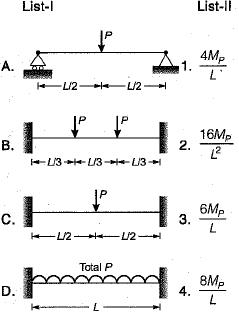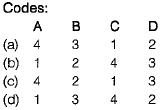Solution: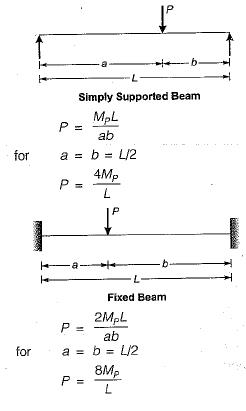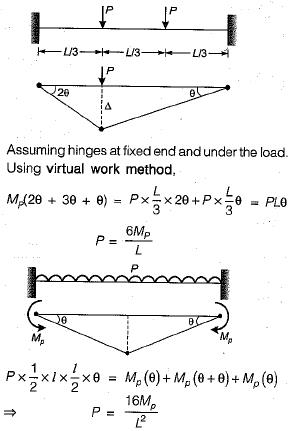QUESTION: 6

Match List-I (Shape of structural) with List-II (Shape factor) and select the correct answer using the codes given below the lists: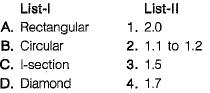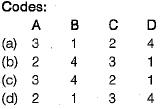Solution:
QUESTION: 7

If a uniform beam shown in the figure below has the plastic moment capacity Mp for span AB and 0.9 Mp for span BC, what is the correct virtual work equation?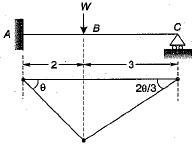Solution:

Total number of plastic hinges needed for complete collapse of the beam may be given as,
Number of plastic hinges,
= r + 1 = (3 - 2) + 1 = 2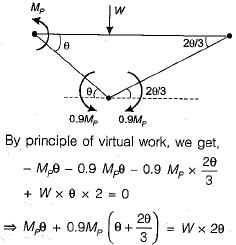QUESTION: 8

What is the number of plastic hinges formed if an indeterminate beam with redundancy R is to become determinate?

Solution:

The number of plastic hinges required to make an indeterminate beam determinate is R where R is the degree of redundancy. However, for the complete collapse of the beam (R + 1) plastic hinges will be required.

QUESTION: 9

The cross-sectional area and plastic section modulus of the given section are respectively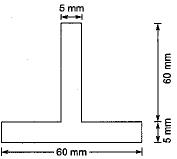Solution:

Total area, A = 5 x 60 + 5 x 60
= 600 mm2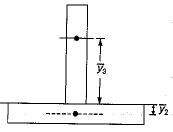The centroids of half areas on either side of axis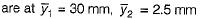Therefore plastic section modulus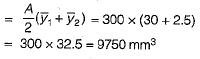QUESTION: 10

A load P is applied at the middle of a simply supported beam of span L. If the beam is made of ductile material, and Mp is the plastic moment, what is the ultimate value of P?

Solution:

The simply supported beam is a determinate one, hence one plastic hinge will be required for its complete collapse. This plastic hinge will be,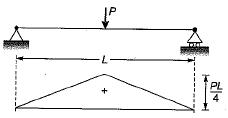formed under the load. Hence the maximum bending moment can at the most reach to the plastic moment Mp.
∴